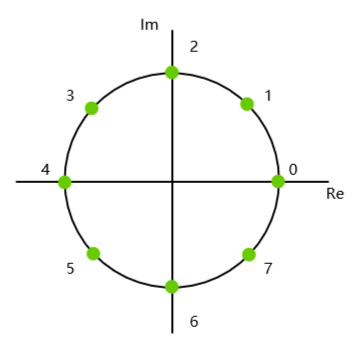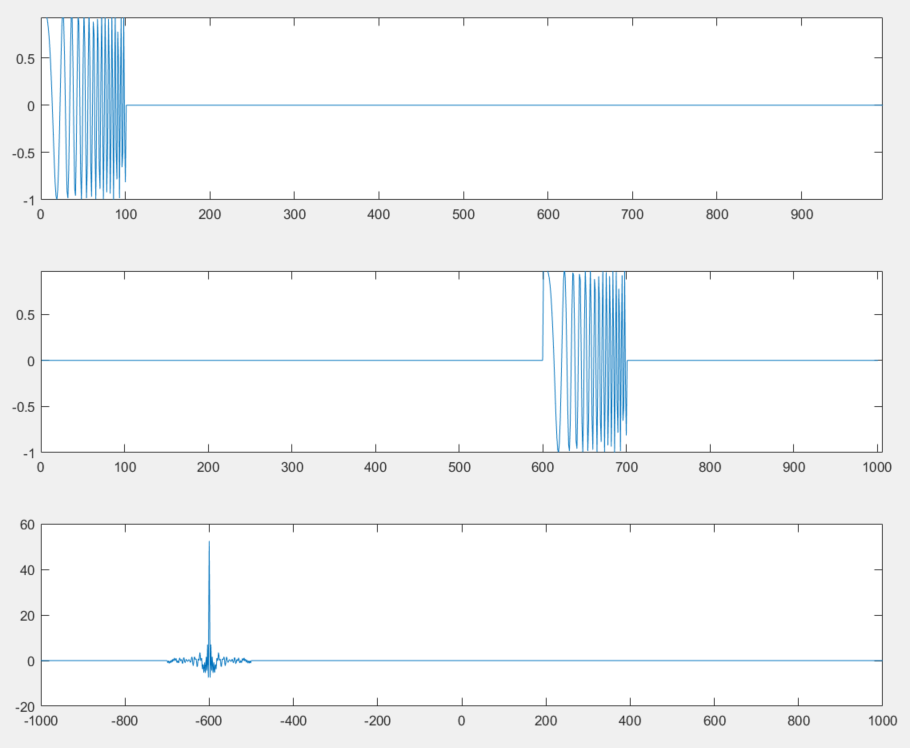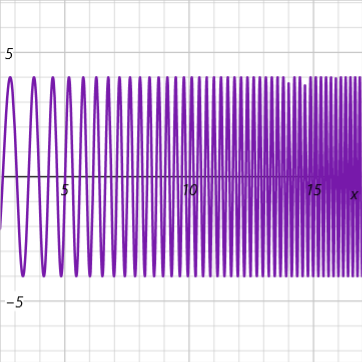• 简单地说，微积分基本定理分为两个部分： 第一部分：d∫fdx = fdx，意思是：函数f积分的微积等于该函数的微分... 据此，微分与积分是互逆运算，也就是说： d∫ = ∫d 这就是全部的微积分学。 从历史发展上来看...
        简单地说，微积分基本定理分为两个部分：        第一部分：d∫fdx = fdx，意思是：函数f积分的微积等于该函数的微分；        第二部分：F = ∫dF，意思是，函数F微分的积分等于该函数的自身。        据此，微分与积分是互逆运算，也就是说：                d∫ = ∫d        这就是全部的微积分学。        从历史发展上来看，积分学先于微分学而存在，16世纪出现微分学之后，牛顿与莱布尼兹将两者联系起来，揭示了两者的本质关系，这是他们的历史贡献。        说明：科普中国把微积分基本定理与牛顿-莱布尼兹“公式”等同起来，不能说明微分与积分是互逆运算的基本事实，也抹杀了牛顿与莱布尼兹对数学的伟大贡献。科普中国啊！袁萌 2月25日
展开全文• 在交换两个int型的变量的数值时通常用到的方法就是引用第三方变量，具体的方法如下：public static void change...}当然也可以通过不引用第三方变量，通过逆运算来实现两个数值的交换。具体方法如下：public stat...
在交换两个int型的变量的数值时通常用到的方法就是引用第三方变量，具体的方法如下：public static void change(int a, int b) {
int temp;   //引用的第三方变量
temp = a;
a = b;
b = temp;
}当然也可以通过不引用第三方变量，通过逆运算来实现两个数值的交换。具体方法如下：public static void change(int a, int b) {
a = a + b;
b = a - b;
a = a - b;
}当然一些水平稍高的人觉得在二进制位上对两个数的数值进行操作耗费资源比较少，而且显得比较高大上，同时异或运算的逆运算还是异或，于是算法可以进一步升级为：public static void change(int a, int b) {
a = a ^ b;
b = a ^ b;
a = a ^ b;
}至此一个优秀的不引入第三方变量的通过位运算交换数值的算法就算是实现了。下面举一个具体的实例：问题：实现对一维数组的倒置。首先我们先用通过引用第三方变量的方式解决：public static void change(int[] a) {
for (int i = 0; i < a.length; i ++) {
int temp = a[i];
a[i] = a[a.length - i - 1];
a[a.length - i - 1] = temp;
}
}通过上述方式很容易就解决了问题，然而结果就是并没有实现数组的倒置，反而和原数组一样。这个方法存在的问题我们暂时先不去深究，我们先看一下用逆运算实现的情况：public static void change(int[] a) {
for (int i = 0; i < a.length; i ++) {
a[i] = a[i] + a[a.length - i - 1];                  //①
a[a.length - i - 1] = a[i] - a[a.length - i - 1];   //②
a[i] = a[i] - a[a.length - i - 1];                  //③
}
}为了便于理解我直接用了加法减法这组互逆运算。如果数组的长度是奇数，无论数组元素的值如何中间的那个元素的值都为0，倒置数组咋还倒置出了0出来了呢？细究原因你就会发现当数组的长度为奇数时，i = a.length / 2 。此刻a[i] = a[a.length - i - 1]，第③步的执行结果直接导致了中间的数字为0。另外，由于当 i >= a.length / 2 之后，前后两部分又重新交换，所以最终除了中间元素变成0以外其他元素的值不会发生变化，这也解释了上一方案为什么原样输出。这个方案用的是加法减法逆运算，我想用其他逆运算虽然不会出现中间元素为零的情况，但一定会有其他异常情况产生。事已至此，我想诸位应该也知道了这个题目的正确解法了，只需在把for循环里的 i < a.length 换成 i < a.length / 2 便可以完美解决。
展开全文• 从傅里叶级数到基于相关运算的声音测距【学习笔记】傅里叶变换傅里叶级数离散傅里叶变换(DFT)快速傅里叶变换(FFT)单位根及其性质单位根如何加速DFT运算 傅里叶变换 傅里叶级数 任何周期函数都可以用正弦函数和余弦...
从傅里叶级数到基于互相关运算的声音测距【学习笔记】傅里叶变换傅里叶级数离散傅里叶变换(DFT)快速傅里叶变换(FFT)单位根及其性质单位根如何加速DFT运算多项式乘法多项式的表示FFT如何加速多项式乘法求值插值卷积与互相关运算卷积互相关运算声音测距
傅里叶变换
傅里叶级数
任何周期函数都可以用正弦函数和余弦函数构成的无穷级数来表示
设一函数周期为$2l$
$f(x) ={a_0\over2}+\sum_{n=1}^\infin a_n cos({n2\pi x\over 2l})+\sum_{n=1}^\infin b_nsin({n2\pi x\over 2l}),n\in N^*$
可以找到一系列特定周期的正余弦函数，使它们互相正交，即乘积在最大周期内的积分为零
$\int_{-l}^lsin({n\pi x\over l})dx = 0;$
$\int_{-l}^lsin({m\pi x\over l})cos({n\pi x\over l})dx = 0,m,n\in N^*;$
$\int_{-l}^lsin({m\pi x\over l})sin({n\pi x\over l})dx = 0,m,n\in N^*;$
……
据此可以以{$1,sin({n\pi x\over l}),cos({n\pi x\over l})$}为基表示出一个周期函数f(x)
求f(x)与每一个基的内积，即相乘求积分，即可得到每个基所对应的系数
$a_0 = {1 \over 2l}\int_{-l}^l f(x)dx,$
$a_n = {1 \over l}\int_{-l}^l f(x)sin({n\pi x\over l})dx,$
$b_n = {1 \over l}\int_{-l}^l f(x)cos({n\pi x\over l})dx,$
${n\pi\over l} = 2\pi f$,若将频率$f$作为自变量，将$a_n,b_n$作为因变量，便可以得到一个频域的图像，有助于我们分析一段信号里的频率特征
下面引入欧拉公式，可以将傅里叶级数写成复数形式：
$f(x) = \sum_{n=-\infin}^{+\infin} c_n e^{i{n\pi x\over l}}$
$c_n ={1\over 2l}\int_{-l}^l f(x)e^{-i{n\pi x\over l}}dx$
对于非周期函数，直接以周期无穷大处理，而对于定义域连续且有限的一段信号，可以以定义域长度为周期，进行傅里叶变换

离散傅里叶变换(DFT)
现实生活中，对信号的数字化采样是离散的，这时就需要离散形式的傅里叶变换（DFT）
主要的改变就是把积分形式改为求和
设有一信号采样频率为$f_s$,采样点数为N，那么对它进行傅里叶变换后可以得到N个频率幅值
$c_n ={1\over N}\sum_{k=0}^{N-1} f(k)e^{i{2\pi n\over N}k}$

通常也把$e^{i{2\pi \over n}k}$记作$\omega_n^k$ , 之后推导FFT的时候会用到
（余以为，指数上的负号应该是用欧拉公式推导复数形式时产生的，这里直接用实部记录余弦分量，以虚部记录记录正弦分量，便省去负号）

对$n\in\{0,1,...,N-1\}$依次计算，对得到的$c_n$取模， 便可以得到一个频率从0~$f_s$的频谱图。自变量频率是等间距分布的，即$c_n$对应的实际频率为$f_s*{n\over N},n\in\{0,1,...,N-1\}$
同时采样定理告诉我们若要完整地保留原始信号中的信息，采样频率应至少大于原始信号中最高频率的两倍。可以只采用前一半结果。（具体原因不懂我瞎说的）
综上，采样频率越高，可以准确测量的频率也就越高（采样频率的一半）；参与DFT运算的采样点越多，频谱的分辨率（$N\over f_s$）就越高，同时也必然导致更长的采样时间和运算时间。
至此已经可以写出离散傅里叶变换(DFT)的代码：
#define N 1024
#define PI 3.1415926535
double x[N];//输入信号
double Re[N],Im[N];//实部虚部分别为cos、sin
double c[N];//输出频谱
int n,k;
for(n=0;n<N;n++)
{
for(k=0;k<N;k++)
{
Re[n] += x[k]*cos(2*PI*k*n/N);
Im[n] += x[k]*sin(2*PI*k*n/N);
}
c[n] = sqrt(Re[n]*Re[n]+Im[n]*Im[n]);//取模
}
这种写法十分简洁易懂，但是很费时。在I.MX RT1064上测试计算1024个采样点需要约1000ms

快速傅里叶变换(FFT)
快速傅里叶变换是一种可以加速离散傅里叶(DFT)运算速度的算法，大大减少了运算量。同时得到傅里叶变换的准确结果。
单位根及其性质
单位根
在复平面的单位圆上取n个点，这些点将圆周n等分。那么它们的指数表示为$e^{i{2\pi \over n}k}，k\in \{1,2,...,n-1\}$，现将它们记为$\omega_n^k，k\in \{1,2,...,n-1\}$，直观来看下标就是圆周被等分的份数，上标是从实轴开始逆时针数过的圆弧个数。显然，这些复数的模长为1，且它们的n次方都为实数1，故称之为单位根。
$\omega_n^k = e^{i{2\pi \over n}k}$
单位根的性质

$\omega_n^k = \omega_{2n}^{2k}$

将圆弧再等分依次，变成2n等分，取2k份圆弧，两者表示同一个复数。也可以直接通过指数形式理解。

$\omega_n^{k+{n\over2}} = -\omega_n^k$

$+{n\over2}$表示在单位圆上逆时针旋转了半圈，即与原点对称，实部虚部取相反数。

$(\omega_n^k)^m = \omega_n^{mk}$

这可以由复数乘法性质得到，模长相乘(=1),幅角相加。

单位根如何加速DFT运算
再贴一下离散傅里叶的公式：
$c_n ={1\over N}\sum_{k=0}^{N-1} f(k)e^{-i{2\pi n\over N}k}$
将$e^{i{2\pi \over n}k}$记为$\omega_n^k$形式：
$c_n ={1\over N}\sum_{k=0}^{N-1} f(k)\omega_N^{nk}$
或$c_n ={1\over N}\sum_{k=0}^{N-1} f(k)(\omega_N^n)^k$
这是我们需要计算的任务。
设$A(x) =\sum_{k=0}^{N-1} f(k)x^k，$并将$f(k)用a_k$表示
则$A(x) = \sum_{k=0}^{n-1}a_kx^k= a_0+a_1x+a_2x^2+...+a_{n-1}x^{n-1}$
这里开始采用分治的思想，按下标奇偶性将数列分成两半（此处规定n为偶数）：
$A(x)=(a_0+a_2x^2+...+a_{n-2}x^{n-2})+(a_1x+a_3x^3+...+a_{n-1}x^{n-1})\\ =(a_0+a_2x^2+...+a_{n-2}x^{n-2})+x(a_1+a_3x^2+...+a_{n-1}x^{n-2})$
令$A_1(x) = (a_0+a_2x+...+a_{n-2}x^{n-2\over2}),\\A_2(x) = (a_1+a_3x+...+a_{n-1}x^{n-2\over2}).$
则$A(x) = A_1(x^2)+xA_2(x^2)$
接下来开始带入单位根$\omega_n^k$(这里令$k<{n\over2}$,分治减少运算量的关键步骤):
$A(\omega_n^k)=A_1((\omega_n^k)^2)+\omega_n^kA_2((\omega_n^k)^2)\\ =A_1(\omega_{n}^{2k})+\omega_n^kA_2(\omega_{n}^{2k})\\ =A_1(\omega_{n\over2}^k)+\omega_n^kA_2(\omega_{n\over2}^k)$
对于$k>{n\over2}$，不妨直接带入$\omega_n^{k+{n\over2}}$(多用单位根的几何意义理解):
$A(\omega_n^{k+{n\over2}})=A_1((\omega_n^{k+{n\over2}})^2)+\omega_n^{k+{n\over2}}A_2((\omega_n^{k+{n\over2}})^2)\\ =A_1(\omega_{n}^{2k+n})+\omega_n^{k+{n\over2}}A_2(\omega_{n}^{2k+n})\\ =A_1(\omega_{n}^{2k}*\omega_n^n)-\omega_n^kA_2(\omega_{n}^{2k}*\omega_n^n)\\ =A_1(\omega_{n}^{2k})-\omega_n^kA_2(\omega_{n}^{2k})\\ =A_1(\omega_{n\over2}^k)-\omega_n^kA_2(\omega_{n\over2}^k)$
观察以上结果，发现前一半($k<{n\over2}$)和后一半的结果十分相似，仅仅差一个符号
根据这个结论，我们就可以只带入前一半单位根的同时得到整个序列的DFT结果
再看两个推导的最后一行，$n写作了{n\over2}$,有了这一步，就可以发现$A_1(\omega_{n\over2}^k)、A_2(\omega_{n\over2}^k)和A(\omega_{n\over2}^k)$其实是等价的，只不过取的单位根少了一半，而这两个一半长度的序列又可以通过上面的分治的方法减半运算……
（典型的递归思想，这时候就需要满足$n = 2^i,i\in N^*$了）
总得来说，FFT是充分利用了复数表示中复数的特性（见单位根的性质），推导出了分治的计算方法，并且可以递归使用，从而大大减少了计算复杂度

递归实现
现在就可以用递归的方式完成FFT的代码：
C语言的复数库对于不同编译器使用不太相同，所以先写一个简单的复数计算需要用到的代码
typedef struct complex_t
{
double x,y;
} complex;

complex plus(complex a,complex b)//+
{
a.x += b.x;
a.y += b.y;
return a;
}

complex minus(complex a,complex b)//-
{
a.x -= b.x;
a.y -= b.y;
return a;
}

complex multi(complex a,complex b)//*
{
complex m;
m.x = a.x*b.x - a.y*b.y;
m.y = a.x*b.y + a.y*b.x;
return m;
}
接下来是递归程序
#define N 1024  //序列总长度，需满足2的整数次幂
#define Pi 3.14159265359

void FFT_recursion(int len,complex *x)//当前序列长度、当前求解序列
{
int i;
complex x1[N],x2[N];	//放按下标奇偶分组的两个序列
complex Wn = {cos(2.0*Pi/len),sin(2.0*Pi/len)};	//k=1单位根，计算A2前系数用
complex w = {1,0},m;	//w用来保存单位根的幂
if(len == 1)return;	//当长度为1时就可以直接返回，什么也不做
for(i=0;i<len;i+=2)		//给半长序列填入相应的值
{
x1[i>>1] = x[i];
x2[i>>1] = x[i+1];
}
FFT_recursion(len>>1,x1);	//求出两个序列分别带入len/2个单位根的值
FFT_recursion(len>>1,x2);	//结果就存储在输入的数组中

for(i=0;i<(len>>1);i++,w = multi(w,Wn))	//这里i相当于公式中的k
{
m = multi(w,x2[i]);	//保存w与A2的乘积
x[i] = plus(x1[i],m);	//同时给前后两部分赋值
x[i+(len>>1)] = minus(x1[i],m);
}
}
如果使用递归写法，就需在调用函数后再对类型为complex的数组进行处理
可以看到这里求的是$A(\omega_n^k)$，要求严谨的幅值还需要再除以N，并且取模方便使用（其实工程中直接除以一个合适的值，方便观察就可以了）
用递归实现的FFT已经比之前的DFT高效很多了，但需要特殊功能的时候还得用另外的函数封装，大数组又占空间，如果能改成用for循环实现岂不美哉？

迭代实现
用递归函数写代码的时候，我们只需要思考其中一步需要完成的操作，以及回归的条件。
那么最终整个递归过程都做了些什么呢，下面我们以N=8为例，把每一步分治的过程写出来。
a0 a1 a2 a3 a4 a5 a6 a7a0 a2 a4 a6a1 a3 a5 a7a0 a4a2 a6a1 a3a5 a7
第一行是原始输入的一组数据，我们要做的是用它们分别乘上$\omega_8^k,k\in[0,7]$然后求和
第二行采用分治方法，按奇偶性分成两列，对它们求值后得到两个长度4的数组，设为A1[ ],A2[ ]，那么用A1±$(\omega_8^1)^0$就可以得到最终需要计算的A和A两个值，其余三对结果求法类似。
下面把第一行和最后一行的下标及其二进制写出来：

原序列
0
1
2
3
4
5
6
7

二进制
000
001
010
011
100
101
110
111

重排后
0
4
2
6
1
5
3
7

二进制
000
100
010
110
001
101
011
111

可以看出排列后递归最底层的顺序是有规律的——下标的二进制表示颠倒
再放一张N=16的表格：

原序列
0
1
2
3
4
5
6
7
8
9
10
11
12
13
14
15

二进制
0000
0001
0010
0011
0100
0101
0110
0111
1000
1001
1010
1011
1100
1101
1110
1111

重排后
0
8
4
12
2
10
6
14
1
9
5
13
3
11
7
15

二进制
0000
1000
0100
1100
0010
1010
0110
1110
0001
1001
0101
1101
0011
1011
0111
1111

下面研究一下如何用程序完成这个排列
（不想研究的可以抄代码，反正就一行 ）
如果把下标的二进制当成数组，一位一位地交换，随着n的增大会越来越麻烦

c语言中右移可以表示整除2，比如5>>1=2, 6>>1=3
一个数（除了二进制最低位）可以看作它整除2的数左移一位，如13(1101)，而6(0110)<<1=12(1100)，前三位和13相同
一个二进制数颠倒后（除了最高位），可以看作它整除2的数也颠倒后右移一位，如13(1101) -颠倒-> 11(1011), 6(0110) -颠倒-> 6(0110) >> 1 = 3(0011)，后三位相同
剩下的一位单独取出（和1作逻辑与运算）进行位移后和其它位作或运算即可
我们只要从0(0本身就对称，移位后也无变化)开始依次操作，每次去取整除2（右移1）的下标所对应的排列后标号，就能把所有排列计算出来

下面是代码：
#define N 1024	//总数据个数
#define L 10	//满足 N = 2^L	//同时也是下标二进制表示的位数

int r[N] = {0};	//可以认为下标是原排列，数据是二进制颠倒后的排列
int i;	//循环变量

for(i=0;i<N;i++)
{
r[i] = (r[i>>1]>>1)|(i&1)<<(L-1); //计算重排后的数组下标
//逻辑或符号左侧是取下标整除2的结果右移一位
//右侧是单独处理剩下来的一位，将下标本身第一位取出，左移L-1位
}
//这部分代码可以直接放进迭代FFT函数中
现在我们已经成功得到了一个排列后的下标，只要按照这个数组给出的顺序循环填入数据就可以开始进行计算了
直接给出完整代码吧：
#define N 1024 //总数据个数
#define L 10 //满足 N = 2^L
#define Pi 3.14159265359

void FFT(complex *x)
{
int r[N] = {0},i,l,j,k;
complex Wn,w;
complex temp,temp1;	//用于交换数组项，以及计算时保存数组原值
for(i=0;i<N;i++)
r[i] = (r[i>>1]>>1)|(i&1)<<(L-1); //计算重排后的数组下标

for(i=0;i<N;i++)	//按r[i]所给顺序重排数组
if(i<r[i])	//防止重复交换
{
temp = x[i];
x[i] = x[r[i]];
x[r[i]] = temp;
}

for(l=1;l<N;l<<=1)	//当前需要计算的数组长度的【一半】，l取1,2,4,8,...,N/2
{
Wn.x = cos(Pi/l);Wn.y = sin(Pi/l);	//准备单位根
for(j=0;j<N;j+=l<<1)	//依次处理每个序列，j每次循环递增2l
{
w.x = 1;w.y = 0;	//记录单位根的幂
for(k=0;k<l;k++,w = multi(w, Wn))	//用上一步算好的值带入公式计算当前序列
{
//由于迭代法只用了一个复数数组，结果也直接记录在本身，
//所以要先用临时变量记录原值。
temp = x[j+k];temp1 = multi(w,x[j+k+l]);
x[j+k] = plus(temp, temp1);
x[j+k+l] = minus(temp, temp1);
}
}
}
}
如需作频谱分析，测得的信号填入数组实部，输出数组进行取模（平方和开根号）即可
实测以上代码在I.MX RT1064上仅需1.5ms！
至此FFT的研究就基本完成了！

多项式乘法
逛了几天，看到最多的对FFT作用的描述就是：加速多项式乘法。
苦恼了好长时间，始终想不通傅里叶级数里哪里用到多项式了。最后才明白，其实是用傅里叶变换的快速算法，去加速多项式的乘法计算。这里已经不谈FFT频域转换的功能了。
多项式的表示
讲多项式乘法还是离不开两种表示方法
系数表示
下面是一个普通的多项式，它有n项，次数是n-1
$f(x) = a_0+a_1x+a_2x^2+...+a_{n-1}x^{n-1}$
把它的系数拿出来，列成一个向量，就得到了多项式的系数表示：
$(a_0,a_1,a_2,...,a_{n-1})$
这是符合我们对多项式的一般认识的表示方法
点值表示
我们选一些x的值带入表达式中，就可以求得多项式的值。如果带入了n个x，得到n个值，理论上就可以通过这一系列方程求解出原式多项式的每一个系数。
设 $yk=f(x_k)$，然后带入$x_k,k\in \{0,1,...,n-1\}$
得$(y_0,y_1,y_2,...,y_{n-1})$
这就是点值表示法
点值表示的多项式作乘法运算时只需要n次乘法。

FFT如何加速多项式乘法
FFT作多项式乘法的过程主要包括求值(DFT)，插值(IDFT) 两部分。
求值指带入x的值，将系数表示转为点值表示；插值指将点值表示转回系数表示。
所以，求两个n项n-1次多项式相乘的结果，首先要对它们分别带入n个x值，求出点值表达（求值），然后循环n次将点值表示相乘，最后把相乘的结果还原为系数表达（插值）。
听起来是不是十分复杂，甚至有点多此一举？而且插值过程可是要求解n元一次方程组啊！
不过要知道，FFT对DFT的加速可不止一倍两倍，而且如果我们求值的过程中选取上述的单位复根作为自变量带入，插值过程就完全不需要解方程！而且与求值过程仅仅一个符号只差（我看呆了 ）！

求值
求值过程与之前讲的FFT类似，同样是带入单位复根$\omega_n^k$，代码完全相同。
$y[k] = \sum_{i=0}^{n-1}x[i](\omega_n^k)^i$
x[ ]为原多项式的系数，y[ ]记录点值表示。而代码中结果直接输出到x[ ]本身。
这里直接调用该函数：
void FFT(complex *x);	//输入复数组，输出求值结果

插值
插值过程与点值过程的区别就在于：

带入自变量时取单位复根的共轭复数 $\omega_n^{-k}$
结果要除以n

插值过程所作的事情见以下表达式：
$c_k = {1\over n}\sum_{i=0}^{n-1}y[i](\omega_n^{-k})^i$
$c_k$为所求得的系数，$y[i]$为已经做过乘法的点值表达
可以看到这里似乎又把已求得的点值表达当成了系数带入求值了，为什么这样做就能解出系数呢？（又要敲公式了，不想看的可以跳过 ）
以下是证明：
设所求多项式的系数表达为$(a_0,a_1,a_2,...,a_{n-1})$，现有的是它经过FFT求值后的点值表达$(y_0,y_1,y_2,...,y_{n-1})$，（其实是通过前面求值的点值多项式相乘得到的）
现设一向量$(c_0,c_1,c_2,...,c_{n-1})$满足$c_k = {1\over n}\sum_{i=0}^{n-1}y_i(\omega_n^{-k})^i$，我们要证明$c_k=a_k$.
首先带入$y_i=\sum_{j=0}^{n-1}a_j(\omega_n^{i})^j$，
$c_k = {1\over n}\sum_{i=0}^{n-1}y_i(\omega_n^{-k})^i\\ ={1\over n}\sum_{i=0}^{n-1}(\sum_{j=0}^{n-1}a_j(\omega_n^{i})^j)(\omega_n^{-k})^i\\ ={1\over n}\sum_{i=0}^{n-1}(\sum_{j=0}^{n-1}a_j(\omega_n^{j})^i)(\omega_n^{-k})^i\\ ={1\over n}\sum_{i=0}^{n-1}(\sum_{j=0}^{n-1}a_j(\omega_n^{j})^i(\omega_n^{-k})^i)\\ ={1\over n}\sum_{i=0}^{n-1}(\sum_{j=0}^{n-1}a_j(\omega_n^{j-k})^i)$
调换求和次序
$c_k={1\over n}\sum_{j=0}^{n-1}a_j(\sum_{i=0}^{n-1}(\omega_n^{j-k})^i)$
当$j=k$时，$\omega_n^{j-k}=1$，$(\sum_{i=0}^{n-1}(\omega_n^{j-k})^i)=n$
当$j!=k$时，记$\omega_n^{j-k}$为$\omega_n^m$
$\sum_{i=0}^{n-1}(\omega_n^{j-k})^i=1+(\omega_n^m)+(\omega_n^m)^2+...+(\omega_n^m)^{n-1}\\ (\omega_n^m)\sum_{i=0}^{n-1}(\omega_n^{j-k})^i=(\omega_n^m)+(\omega_n^m)^2+(\omega_n^m)^3+...+(\omega_n^m)^{n}$
做差，得
$(\omega_n^m-1)\sum_{i=0}^{n-1}(\omega_n^{j-k})^i=(\omega_n^m)^{n}-1$
$\sum_{i=0}^{n-1}(\omega_n^{j-k})^i={(\omega_n^m)^{n}-1\over\omega_n^m-1}$
注意$(\omega_n^m)^{n}=1$
所以$\sum_{i=0}^{n-1}(\omega_n^{j-k})^i=0$
再看上面的式子：
$c_k={1\over n}\sum_{j=0}^{n-1}a_j(\sum_{i=0}^{n-1}(\omega_n^{j-k})^i)\\ ={1\over n}a_k(\sum_{i=0}^{n-1}(\omega_n^{0})^i)\\ ={1\over n}a_k*n\\ =a_k$

证明完毕，可以开始写代码了！
#define N 8	//大于等于项数和且满足2的整数次幂
#define L 3

double* FFT_Convolution(double *a, double *b)
{
int r[N] = {0},i,j,l,k;
complex x[N],y[N];
complex w,Wn,x1,x2,y1,y2;
for(i=0;i<N;i++)
{
r[i] = (r[i>>1]>>1)|(i&1)<<(L-1); //计算重排后的数组下标
x[i].x = a[r[i]];x[i].y = 0.0;	//按r[i]重排x,y
y[i].x = b[r[i]];y[i].y = 0.0;
}
//数组次序排列完成
for(l=1;l<N;l<<=1)  //求值
{
Wn.x = cos(Pi/l);Wn.y = sin(Pi/l);
for(j=0;j<N;j+=l<<1)
{
w.x = 1.0;w.y = 0.0;
for(k=0;k<l;k++,w = multi(w, Wn))
{
x1 = x[j+k];
y1 = y[j+k];
x2 = multi(w,x[j+k+l]);
y2 = multi(w,y[j+k+l]);
x[j+k] = plus(x1, x2);     //同时操作两个序列
x[j+k+l] = minus(x1, x2);
y[j+k] = plus(y1, y2);
y[j+k+l] = minus(y1, y2);
}
}
}

for(i=0;i<N;i++)    //求积
y[i] = multi(x[i],y[i]);

for(i=0;i<N;i++)	//再次重拍
x[i] = y[r[i]];

for(l=1;l<N;l<<=1)  //插值
{
Wn.x = cos(Pi/l);Wn.y = -sin(Pi/l);	//这里多了一个负号
for(j=0;j<N;j+=l<<1)
{
w.x = 1.0;w.y = 0.0;
for(k=0;k<l;k++,w = multi(w, Wn))
{
x1 = x[j+k];
x2 = multi(w,x[j+k+l]);
x[j+k] = plus(x1, x2);
x[j+k+l] = minus(x1, x2);
}
}
}

for(i=0;i<N;i++)	//将结果填入数组b
b[i] = x[i].x/N;

return b;
}

需要注意的是N必须要大于等于所输入的两个多项式项数和

卷积与互相关运算
现在我们已经能求出多项式相乘的结果了，那么它有什么用呢？显然不仅仅是求多项式。
卷积
当我们平时手算多项式乘法时，如果次数比较高，求出来的项会特别多，所以我一般会按次数顺序计算，即先从0次项，再去找1次项的系数，以此类推。
如果把每一项的系数按顺序写出来，就会发现只要把两个系数相向放置，然后一项一项错开，把对应的系数相乘再求和就可以得到该次数的项的系数。
$a_3,a_2,a_1,a_0..............\\...............b_0,b_1,b_2,b_3$$a_0*b_0=c_0（常数项系数）$
$a_3,a_2,a_1,a_0..........\\.........b_0,b_1,b_2,b_3$$a_1*b_0+a_0*b_1=c_1（一次项系数）$
$a_3,a_2,a_1,a_0....\\.....b_0,b_1,b_2,b_3$$a_2*b_0+a_1*b_1+a_0*b_2=c_2（二次项系数）$
$......$
即$c_k=\sum_{i=0}^{k}a_i*b_{k-i}$
这个操作就是卷积
卷积在工程信号处理中有着广泛的应用，遇到卷积时，我们就可以用上述FFT算法来计算。
double* FFT_Convolution(double *a, double *b);

互相关运算
与卷积的区别在于第一个序列不需要逆序
将序列$\{a_n\}$固定，移动$\{b_n\}$，从-(n-1)到(n-1)
最直观的物理意义就是经过位移，两个序列中相似的部分重叠的时候，得到的运算结果最大。由此可以作信号的识别。
在MATLAB中的函数为xcorr(x,y);
在上述卷积代码中只需在数组b赋值时反向填入即可
下面是一个例子，可以明显看出第二个序列向左移动600个单位时和第一个序列最相似（这里是重叠）声音测距
下图是一段Chirp信号A chirp is a signal in which the frequency increases (up-chirp) or decreases (down-chirp) with time.
Chirp信号是一段频率随时间增加或降低的信号。

为什么用Chirp信号测距呢？
声音测距的基本原理就是测量声音在空气中传播的延迟，通过发出一段Chirp信号，再去对它进行采集，通过与原信号进行互相关运算就可以知道它延迟的时间，从而算出距离。
那为什么不直接判断相位差呢？可以简单的计算一下，常温中声速约为334m/s，假设声音信号传播10米后被接收，这期间过去了大约30ms，而一个440hz的声音信号已经走过了13个周期，这中间跳过的周期是我们无法测量的。
而使用Chirp信号，我们可以按需设置它一个周期的时长，就可以避免这个问题，而且调频信号一个周期内的特征点比一个简单的正弦波要多得多，测量也就更加精确。

为了得到精确的时间延迟，也就是希望信号相关结果出现的峰值约尖锐越好，作为测距的声音信号需要频谱较宽，比如时间很短的脉冲信号、具有变频的Chirp信号、白噪声信号等等。利用声音定位的动物们常常使用的是Chirp信号。

采样完整周期的声音信号后，和原式数据作互相关运算，得到峰值处的横坐标，乘上采样周期即可得到声音传播延迟，再乘上声速即可算出距离。

终于终于结束了！（累die ）
这一周在各大论坛逛了无数篇有关傅里叶的博客，但奈何数学已经还了好多给老师，废了很大的劲才勉强理解。得出的结论是，每个人自身薄弱的地方不同，学习时着重的点也不同，写的东西大多是针对自身认为的难点。于是决定自己针对我理解中的难点，结合自己的理解写一篇笔记，以便日后查阅。（如果能帮到大家理解就更好啦）
其中一些内容包含自身理解，可能有错误或者不严谨的地方，查阅时要注意。
下面贴上主要参考的博文：

傅里叶级数 三角形式 到 复数形式
离散傅里叶变换-DFT（FFT基础）
FFT频谱分析原理
FFT算法讲解——麻麻我终于会FFT了！
快速傅里叶变换(FFT)详解
十分简明易懂的FFT（快速傅里叶变换）

——2020/3/25


展开全文算法 卷积 信号处理 fft
• 单目运算符“&”是取操作对象的地址，“*”是取指针指向的对象的内容，两个互为互逆运算。 示例：int a = 10,*p; p = &a;//p指针指向了a所在的地址，&a就是将a所在的地址取出来； *p = 90;//p指向的地址内容由10...
说明：本文主要阐述指针的基本运算及算术运算，指针与数组的关系，指针与字符串的关系。

指针运算

1.指针的基本运算包括取地址以及取值运算等运算。
单目运算符“&”是取操作对象的地址，“*”是取指针指向的对象的内容，两个互为互逆运算。
示例：

int a = 10,*p;
p = &a;//p指针指向了a所在的地址，&a就是将a所在的地址取出来；
*p = 90;//p指向的地址内容由10修改为90，这时a等于90；

2.指针的算术运算
指针的算术运算与其基类型有关，所谓的基类型就是所定义的变量的类型。
示例：

#include <stdio.h>

int main() {

double a = 10.6;//double类型的a，基类型为double，占8个字节地址
int b = 89.5;//int类型的b，基类型为int，占4个字节地址

double *pa = &a;//指针赋值
int *pb = &b;

printf("pa = %p\n",pa);//打印初始地址
printf("pb = %p\n",pb);

pa += 2;//指针变量加2
pb += 2;

printf("pa = %p\n",pa);//打印加1后的地址
printf("pb = %p\n",pb);

return 0;
}

运行结果：

pa = 0x7fff5fbff750
pb = 0x7fff5fbff74c
pa = 0x7fff5fbff760
pb = 0x7fff5fbff754

从运行结果可以看出，pa指针在加2运算后，地址增加了16，而pb加2运算后指针所指向的地址增加了8，这是为什么呢？没错，就是加上了基类型所占的字节数乘以n。假设指针所指向的地址为a，在进行加n运算后，所指向的地址为基类型所占字节*n再加上a，指针减法运算同理。

3.指针与数组

先定义一个数组：int array = {3,5,6,7};
在这里，array为数组名，在计算机中代表的是该数组的首地址，即*array会等于3；这里array可以看做是一个常量，不可以使用自加之类的运算。
再定义一个指针变量int *parr; parr = array;parr是一个指针变量，parr = array的含义为将数组array的首地址赋值给parr值，现在我们可以通过parr指针就可以访问整个array数组，但是parr指针本质并没有改变，依然是一个指针变量，它不能够代表整个数组的内存，相当于是array的一个代表，可以处理数据，但是没有所有权。由于parr依然是指针变量，所以我们可以对它进行自加之类的运算，例如：parr++;自加之后parr指针指向了&array;也可以通过parr指针访问数组里面的元素，例如：*parr;

看文字不爽？上代码：

#include <stdio.h>

int main() {

int array = {3,5,6,7};

printf("%d\n",*array);//打印3

//array++;//编译不通过，会报错,array是常量，不能够执行自加运算

int *parr = array;//array数组的代表登场

*parr = 8;//代表说我有权更改数据
printf("%d\n",array);//打印8

parr++;//由于代表是指针类型，有自己的特殊功力，开始自加
printf("%d\n",*parr);//5
printf("%d\n",array);//5

return 0;
}

总结

1.指针在C语言中可以灵活的运用；
2.数组名为数组所存储空间的首地址。

练习

1.求下面程序的运行结果？为什么？答案

#include<stdio.h>

int main(void){
int a={1,2,3,4,5};

int *ptr=(int *)(&a+1);

printf("%d,%d\n",*(a+1),*(ptr-1));

return 0;
}
展开全文c语言 指针 数组
• 前缀和与差分是一对互逆运算，差分序列B的前缀和序列就是原序列A，前缀和序列的差分序列也是原序列A
• 算术//方法1：加减互逆运算 int a=5,b=3;//需要交换的两个变量 a=a+b;b=a-b;a=a-b;/*方法2：乘除互逆运算 *（因除法可能获得不了精确结果，且比加减法易进位，容易溢出；所以不推荐此种）*/int c=5,d=3;//需要交换的...
• /* 每一堆的数值与ans相异或，所得的结果就是这一堆可以取的数量... 异或运算本身就是互逆运算 */ #include <iostream> using namespace std; int value; int main (){ int n,sum,count,i; w...
• /* Str ：需要处理的字符串(长度为Len) Suffix[i] ：Str下标为i ... Suffix[SA[Len]],即排名为i的后缀为Suffix[SA[i]] (与Rank是互逆运算) 最长公共前缀（Longest Common Prefix，LCP） Heigth[i] : 表示Suffix[SA[i
• 问题1：如何不使用中间变量来实现两个整形变量的交换。解决这个问题是思路很多，首先可以用加...其实任何满足互逆运算的运算都可以用来实现两个整形变量的交换。异或运算和乘除运算都可以实现两个整形变量的交换。 ...
• 我也不知道为什么我不会的大多是基础算法… 定义 对于一维来说，前缀和与差分的处理...由此可以看出，前缀和和差分是一对互逆运算。 差分序列B的前缀和序列就是原序列A 前缀和序列S的差分序列就是原序列A 上...
• 并且其为互逆运算 导数 导数的定义 导数（Derivative），也叫导函数值。又名微商，是微积分中的重要基础概念。当函数y=f(x)的自变量x在一点x0上产生一个增量Δx时，函数输出值的增量Δy与自变量增量Δx的比值在Δx...
• 191114-差分 定义 对于一个给定的序列A，它的差分序列B定义为：B1=A1,Bi=Ai...1，容易发现，前缀和与差分是一对互逆运算，前缀和序列的差分序列就是原序列，差分序列的前缀和序列就是原序列 2，把A序列中[l,r][l,r][...
• 在一定程度上，我们可以将积分和求导当成互逆运算。 可是如果为变限积分也即在积分上下限中也存在变量的情况下，就不是简单地将积分号去掉这么简单了，该如何运算呢。 一般教辅书中会给出这个公式 (∫ϕ1(x)ϕ2(x)f...
• 1数学分析中有积分与微分的互逆运算: \beex \bea f\in R[a,b]&\ra f\ae \mbox{ 连续}\\ &\ra\frac{\rd }{\rd x}\int_a^x f(t)\rd t =f(x),\ae\\ &\ra \mbox{积分后微分可 }\ae\mbox{ 还原};\\ f'\in R...
•   容易发现，“前缀和” 和 “差分” 是一对互逆运算，差分序列B的前缀和序列就是原序列A，前缀和序列S的差分序列也是原序列A。   把序列A的区间 [l,r] 加上 d（即把 Al，Al+1……Ar 都加上 d），其差分序列 B ...
• 按位取反再加1是一个互逆运算。a取反加一，再取反加一，还等于a ------------------------------------ 在计算机中，减法可以用加法来代替，用的就是补码。说道补码，就得说道“模”这个概念。假如我有一个计算机...
• 运算【转载】  转载博主：Matrix67 转载地址：Matrix67的位运算四连发 什么是位运算？  程序中的所有数在计算机内存中都是以二进制的形式储存的。位运算说穿了，就是直接对整数在内存中的二进制位进行...
• 四则运算互逆关系 1 加法与减法 2 乘法与除法 3.估算方法 知识基础练 一填空题 3 3 2 么比少 二判断 一个四位数除以两位数商可能是两 位数也可能是三位数 两数相乘积一定大于第一个因数 0.70.2=3
• 间接存取运算： & 取地址运算符 * 取值运算符二者可以看作一对互逆运算符。在指针定义的时候“表示”“指向”， 在使用指针运算的时候，“*”表示取该指针变量所指向变量的值。 例如： int n =2, * p; p = &n; &(*...
• 第九章 指针 内存地址简称为地址 内存中存放的内容统称... 互逆法则&与*是一对互逆运算符 指针的四要素 指针的赋值运算 指针的初始化 指针与整数的加减运算 指针的自增自减运算 同类指针的相减运算 同类指针的关系运算
• PAGE 精选 数的运算运算的意义 PAGE 精选 金钥匙 四则运算的意义 加法的意义 减法的意义 乘法的意义 除法的意义 四则运算互逆关系 加法与减法 乘法与除法 3.估算方法 知识基础练 一填空题 1 EQ \F(3,4) 3表示 EQ \...
• 有关模运算 定义 运算规则 逆元 定义 使用方法 求逆元的方法 枚举法 拓展欧几里得(Extend - Eculid) 费马小定理(Fermat's little theorem) 注意 有关模运算 在信息学竞赛中，当答案过于庞大的时候，我们...费马小定理
• 矩阵运算 1. 矩阵与数相乘。与矩阵每个元素相乘 2. 矩阵加减。对应位置元素相加减。shape属性必须一致 3. 矩阵相乘。m行n列 乘 n行l列 等于 m行l列。推荐使用matmul，dot 4. 矩阵对应元素相乘。用multiply 5. m.H...矩阵乘法 共轭矩阵 转置矩阵
• 数乘矩阵矩阵的加法运算矩阵乘法运算矩阵运算基本性质单位阵矩阵转置矩阵正交矩阵秩线性方程组高斯消元舒尔补LU分解坐标空间的变换 一个m×n矩阵 当m=n时，A叫做n阶方阵(或n阶矩阵)。 只有一行的矩阵...
• c基本数据类型及其运算第2章 数据类型及其运算 程序由算法和数据构成。 数据是算法的处理对象。 要学习程序设计，首先要了解处理对象—数据的特点。本章中我们讨论C语言中基本数据及其类型和基本的运算方法。 Using ...
• TP5 实现运算验证码 1，直接修改topthink-Captcha中的验证码类captcha.php。 <?php // +---------------------------------------------------------------------- // | ThinkPHP [ WE CAN DO IT JUST THINK ...tp5...